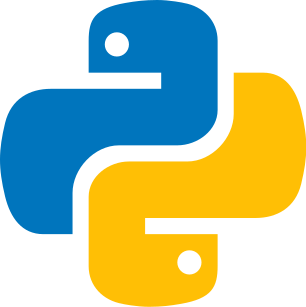Codistwa

# Learn Functions in Math

## with Numpy

Last update : February 11th, 2022

To define a function is to establish a link, a relation between a first set and another set.

In mathematics, a function allows defining a result (most often numerical) for each value of a set called a domain.### Definition of fonction

We talk about image and argument. A number has a unique image, but a number can have several arguments.

Here the argument is the input data, and the image is the returned result.

#### Codepython
1def area(x):
2    return 2 * x
3
4print(area(4))
5
6x = np.linspace(-6,6)
7f = x*2
8
9plt.plot(x,f,label='f')
10
11plt.axis([0,6,0,6])
12plt.grid()
13plt.legend()
14
15plt.title('Function',fontsize=12)
16plt.savefig('function', bbox_inches='tight')

Time complexity : linear

#### FormulaA function in mathematics allows defining a result for each value of a set called a domain.